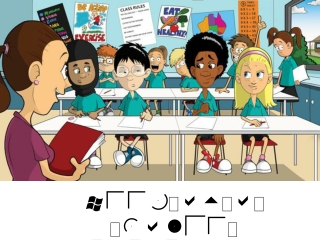DownloadDownload PresentationWeek at a Glance September 16-19

# Week at a Glance September 16-19

Télécharger la présentation## Week at a Glance September 16-19

- - - - - - - - - - - - - - - - - - - - - - - - - - - E N D - - - - - - - - - - - - - - - - - - - - - - - - - - -
##### Presentation Transcript

1. Week at a Glance September 16-19

3. Dear Parents, This week at a glance (WAG) is designed to give parents a brief idea of what their child is learning at school. It will have the subject standards (Georgia Standards of Excellence), the main topics covered, and some sample problems with links to videos and worksheets. We encourage parents and students to review this presentation together and to stay informed on the weekly learning objectives. *** students can earn extra credit if they copy or print the sample problems or worksheets and show the teacher. If the worksheet or PDF has several pages (5 or more), you don’t have to print every page. Print at your own discretion.

4. In an effort to increase our student and parental use of our WAG’s, we will be offering various incentives for the month of September and October. Go to page 10, either print it or student will have to copy it on a sheet of paper. Submit to your teacher by 9/20/19. Extra computer time Homework pass Sit by friend Use technology during lunch Extra credit toward class assignment

5. In an effort to incorporate technology in the classroom and to increase math practice outside the classroom, I’m asking parents to consider adding a couple math learning apps to their child’s phone. iTooch 7th Grade - Math Math Champ Challenge Math Practice - Integers Some apps are free but other range from \$0.99 to \$5.99

6. Our Weekly Focus this week will be Multiplying Integers Dividing Integers Multiply and Divide Rational numbers Divide rational numbers + - x / Fractions Integers (positive and negative numbers)

7. MGSE7.NS.2c Apply properties of operations as strategies to multiply and divide rational numbers. MGSE7.NS.2d Convert a rational number to a decimal using long division; know that the decimal form of a rational number terminates in 0s or eventually repeats Vocabulary: Absolute value, Additive inverse, Expression, Integers, Difference, Repeating decimal, Opposites, Product, Sum, Quotient, Divide, Dividend, Divisor, Terminating decimal MGSE7.NS.2c Apply properties of operations as strategies to multiply and divide rational numbers. MGSE7.NS.2d Convert a rational number to a decimal using long division; know that the decimal form of a rational number terminates in 0s or eventually repeats Vocabulary: Absolute value, Additive inverse, Expression, Integers, Difference, Repeating decimal, Opposites, Product, Sum, Quotient, Divide, Dividend, Divisor, Terminating decimal MGSE7.NS.2c Apply properties of operations as strategies to multiply and divide rational numbers. MGSE7.NS.2d Convert a rational number to a decimal using long division; know that the decimal form of a rational number terminates in 0s or eventually repeats Vocabulary: Absolute value, Additive inverse, Expression, Integers, Difference, Repeating decimal, Opposites, Product, Sum, Quotient, Divide, Dividend, Divisor, Terminating decimal MGSE7.NS.2c Apply properties of operations as strategies to multiply and divide rational numbers. MGSE7.NS.2d Convert a rational number to a decimal using long division; know that the decimal form of a rational number terminates in 0s or eventually repeats. Vocabulary: Absolute value, Additive inverse, Expression, Integers, Difference, Repeating decimal, Opposites, Product, Sum, Quotient, Divide, Dividend, Divisor, Terminating decimal MGSE7.NS.2c Apply properties of operations as strategies to multiply and divide rational numbers. MGSE7.NS.2d Convert a rational number to a decimal using long division; know that the decimal form of a rational number terminates in 0s or eventually repeats. Vocabulary: Absolute value, Additive inverse, Expression, Integers, Difference, Repeating decimal, Opposites, Product, Sum, Quotient, Divide, Dividend, Divisor, Terminating decimal

8. Absolute value Additive inverse Expression Integers Difference Repeating decimal Opposites Product Sum Quotient Divide Dividend Divisor Terminating Decimal

9. Grade Recovery Every week students will get their quiz or test back to see their score. All student are required to write down the problems they missed. If a student received a score below 75, they will correct all mistakes and re-submit as “Grade Recovery”. In addition to correcting their quiz or test, they will also be required to complete at least 30 minutes of i-Ready Math at home. The grade recovery paper must have a teacher and parent signature.

10. Print or copy this page Multiplying and Dividing Integers Rules for Multiplying and Dividing Integers 1. The product or quotient of a positive and negative integer is a negative integer. 2. The product or quotient of two negative or two positive integers is a positive integer. 3. If there are more than two integers, count the number of negative signs: a. If there is an even number of negative signs, the answer is positive. b. If there is an odd number of negative signs, the answer is negative. Multiply or Divide. 1. -3 * -6 2. 15 * -3 3. -4 * 9 4. -3 * -5 * -6 5. -24 ÷ -3 6. 40 ÷ -8 7. 10 * -9 8. -98 ÷ 7 9. -36 ÷ -3 10. -45 ÷ -3 ÷ 5 11. 9 * -12 ÷ 4 12. -5 * 25 13. -7 * -15 14. 65 ÷ -5 15. -5 * -4 * -12 16. 96 ÷ -3 Click link to open document https://www.okaloosaschools.com/pryor/sites/okaloosaschools.com.pryor/files/users/canasylvester/Multiplying%20&%20Dividing%20Integers%20Support.pdf

11. Math Antics - Long Division with 2-Digit Divisors https://www.youtube.com/watch?v=HdU_rf7eMTI

12. Math Antics - Integer Multiplication & Division https://www.youtube.com/watch?v=K_tPbVPfHgk

13. Week 1-5 Resources

14. Math Resources Great Website with Resources: Mrs. Dorset’s Website Weebly http://dorsetw.weebly.com/unit-2---integers.html Describing Integers: this is a PDF https://www.gisd.org/cms/lib/TX01000788/Centricity/Domain/88/Unit_4_Handouts_B.pdf